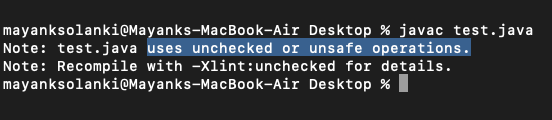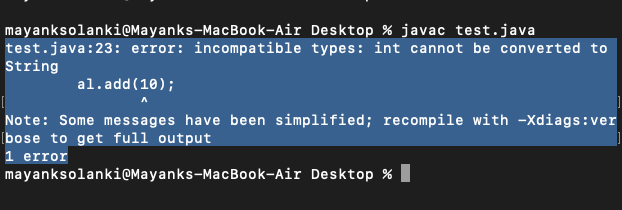# Non-generic Vs Generic Collection in Java

We will be discussing differences later prior let us understand what is generic Collection and non-generic Collection, and most importantly dealing with the implementation part as during implementation one can only really get the real understanding of the concept, henceforth the differences between them.

Generics are basically the errors appearing are compile-time than at run-time. there are certain advantages of generics over non-generic are as follows:

1. Code Reuse: With help of Generics, one needs to write a method/class/interface only once and use it for any type whereas, in non-generics, the code needs to be written again and again whenever needed.
2. Type Safety: Generics make errors to appear compile time than at run time (It’s always better to know problems in your code at compile time rather than making your code fail at run time).

Example: To create an ArrayList that store name of students and if by mistake programmer adds an integer object instead of string, the compiler allows it. But, when this data is retrieved from ArrayList, it causes problems at runtime for Non-generic ArrayList

Implementation:

Example 1

## Java

 `// Java program to Demonstrate that Not Using Generics` `// Can cause Run Time Exceptions`   `// Importing all utility classes` `import` `java.util.*;`   `// Main class` `class` `GFG {`   `    ``// Main driver method` `    ``public` `static` `void` `main(String[] args)` `    ``{` `        ``// Creating an ArrayList` `        ``// Declaring object without any type specified` `        ``ArrayList al = ``new` `ArrayList();`   `        ``// Adding elements to the above object` `        ``// Custom input elements` `        ``al.add(``"Sachin"``);` `        ``al.add(``"Rahul"``);`   `        ``// Compiler will allows this operation` `        ``al.add(``10``);`   `        ``String s1 = (String)al.get(``0``);` `        ``String s2 = (String)al.get(``1``);`   `        ``// Try block to check for exceptions` `        ``try` `{`   `            ``// Causes Runtime Exception` `            ``String s3 = (String)al.get(``2``);` `        ``}`   `        ``// Catch block to handle the exceptions` `        ``catch` `(Exception e) {`   `            ``// Display the exception` `            ``System.out.println(``"Exception: "` `+ e);` `        ``}` `    ``}` `}`

Output:

```prog.java:19: warning: [unchecked] unchecked call to add(E) as a member of the raw type ArrayList
^
where E is a type-variable:
E extends Object declared in class ArrayList
prog.java:20: warning: [unchecked] unchecked call to add(E) as a member of the raw type ArrayList
^
where E is a type-variable:
E extends Object declared in class ArrayList
prog.java:23: warning: [unchecked] unchecked call to add(E) as a member of the raw type ArrayList
^
where E is a type-variable:
E extends Object declared in class ArrayList
3 warnings```How generics solve this problem?

If this list was made Generic, then it would take only String objects and threw Compile Time Error in any other case.

Example 2

## Java

 `// Java Program to Illustrate Conversion of` `// Runtime Exceptions into compile time errors` `// Using generics`   `// Importing all utility classes` `import` `java.util.*;`   `// Main class` `class` `GFG {`   `    ``// Main driver method` `    ``public` `static` `void` `main(String[] args)` `    ``{` `        ``// Creating an ArrayList` `        ``// Declaring object of string type` `        ``ArrayList al = ``new` `ArrayList();`   `        ``// Adding elements to the ArrayList` `        ``// Custom input elements` `        ``al.add(``"Sachin"``);` `        ``al.add(``"Rahul"``);`   `        ``// Now compiler does not allow this operation` `        ``al.add(``10``);`   `        ``String s1 = al.get(``0``);` `        ``String s2 = al.get(``1``);` `        ``String s3 = al.get(``2``);` `    ``}` `}`

Output:

```prog.java:24: error: incompatible types: int cannot be converted to String
^
Note: Some messages have been simplified; recompile with -Xdiags:verbose to get full output
1 error```

Now moving forward, Individual Type Casting is not needed.

If Generics is not needed, then, in the above example every time the data is to be retrieved from ArrayList, it needs to be typecasted. Typecasting at every retrieval operation is a big headache. This can be avoided if somehow it is already known that the list only holds string data.

Example 3

## Java

 `// Java program to Illustrate Type Casting is Needed` `// Everytime in Non-Generic`   `// Importing all utility classes` `import` `java.util.*;`   `// Main class` `class` `GFG {`   `    ``// Main driver method` `    ``public` `static` `void` `main(String[] args) {`   `        ``// Creating an ArrayList` `        ``// Declaring object without any type specified` `        ``ArrayList al = ``new` `ArrayList();`   `        ``// Adding elements to the above object` `        ``// using add() method` `        ``al.add(``"Sachin"``);` `        ``al.add(``"Rahul"``);`   `        ``// For every retrieval,` `        ``// it needs to be casted to String for use` `        ``String s1 = (String)al.get(``0``);` `        ``String s2 = (String)al.get(``1``);` `    ``}` `}`

Output:Geek, now you should be wondering how generics solve this problem?

If this list was made Generic, then it would take only String objects and would return only String objects while retrieval. And hence individual typecasting won’t be required. The above statement is justified

Example 4

## Java

 `// A Simple Java program to demonstrate that` `// type casting is not needed in Generic`   `import` `java.util.*;`   `class` `Test {` `    ``public` `static` `void` `main(String[] args)` `    ``{` `        ``// Creating an ArrayList` `        ``// Declaring object of type String   ` `        ``ArrayList al = ``new` `ArrayList();`   `        ``// Custom input elements ` `        ``al.add(``"Sachin"``);` `        ``al.add(``"Rahul"``);`   `        ``// Retrieval can be easily` `        ``// without the trouble of casting` `        ``String s1 = al.get(``0``);` `        ``String s2 = al.get(``1``);` `      `  `        ``// Print and display out the elements in objects ` `        ``System.out.print(al);` `    ``}` `}`

Output

`[Sachin, Rahul]`

Note:

With the help of generics, while one can implement algorithms Implementing generic algorithms, one can have that work on different types of objects and at the same they are type-safe too.

Do remember that there are some points, which will describe the difference between Generics and Non-Generic which are tabulated below in order to get a crisp understanding between them.

Whether you're preparing for your first job interview or aiming to upskill in this ever-evolving tech landscape, GeeksforGeeks Courses are your key to success. We provide top-quality content at affordable prices, all geared towards accelerating your growth in a time-bound manner. Join the millions we've already empowered, and we're here to do the same for you. Don't miss out - check it out now!

Previous
Next